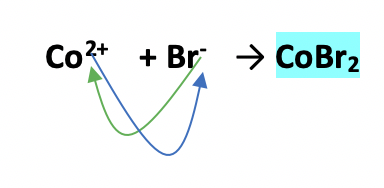# Problem: Determine the chemical formula of each of the following compounds and then use it to calculate the mass percent composition of each constituent element.cobalt(II) bromide

###### FREE Expert Solution

From the name cobalt (II) bromide,

• we have bromine as the anion with a charge of -1, Br-.
• we have cobalt, transition metal as the cation; based on the given name, it has a charge of +2, Co2+.

The chemical formula is:Step 1. Compute for the mass of each element for every 100 sample.

26.94 g Co

73.06 g Br

Step 2. Calculate percent composition.###### Problem Details

Determine the chemical formula of each of the following compounds and then use it to calculate the mass percent composition of each constituent element.

cobalt(II) bromide